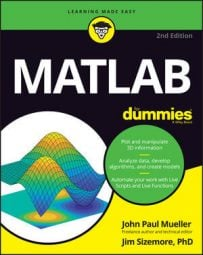##### MATLAB For DummiesMATLAB provides a number of ways in which to create multidimensional arrays. The first method is to simply tell MATLAB to create it for you and fill each of the elements with zeros.

## Creating a multidimensional matrix

The zeros() function helps you perform this task. To create a 2 x 3 x 3 matrix, you type aj = zeros(2, 3, 3) and press Enter. You see the following output:

```aj(:,:,1) =
0 0 0
0 0 0
aj(:,:,2) =
0 0 0
0 0 0
aj(:,:,3) =
0 0 0
0 0 0```

This output tells you that there are three stacked 2 x 3 matrices and each one is filled with zeros. Of course, you might not want to start out with a matrix that’s filled with zeros, so you can use another approach. The following steps help you create a 2 x 3 x 3 matrix that is already filled with data:

1. Type ak(:,:,1) = [1, 2, 3; 4, 5, 6] and press Enter.

You see the following result:

```ak =
1 2 3
4 5 6```

This step creates the first page of the three dimensional matrix. You want three pages, so you actually need to perform this step three times.

2. Type ak(:,:,2) = [7, 8, 9; 10, 11, 12] and press Enter.

MATLAB adds another page, as shown:

```ak(:,:,1) =
1 2 3
4 5 6
ak(:,:,2) =
7 8 9
10 11 12```

If you look at the Workspace window at this point, you see that the size column for ak is now 2 x 3 x 2. It’s at this point that you see the third dimension added. Before you added this second page, MATLAB simply treated ak as a 2 x 3 matrix, but now it has the third dimension set.

3. Type ak(:,:,3) = [13, 14, 15; 16, 17, 18] and press Enter.

The output now looks much like the aj output, except that the elements have values, as shown here:

```ak(:,:,1) =
1 2 3
4 5 6
ak(:,:,2) =
7 8 9
10 11 12
ak(:,:,3) =
13 14 15
16 17 18```

You don’t have to define assigned values using multiple steps. The cat() function lets you create the entire three-dimensional matrix in one step. The first entry that you make for the cat() function is the number of dimensions. You then add the data for each dimension, separated by commas.

To see how this works, type al = cat(3, [1, 2, 3; 4, 5, 6], [7, 8, 9; 10, 11, 12], [13, 14, 15; 16, 17, 18]) and press Enter. You see this output (which looks amazingly like the ak matrix):

```al(:,:,1) =
1 2 3
4 5 6
al(:,:,2) =
7 8 9
10 11 12
al(:,:,3) =
13 14 15
16 17 18```

You may also decide that you don’t want to type that much but still don’t want zeros in the matrix. In this case, use the randn() function for random normally distributed data or the rand() function for uniformly distributed data. This function works just like the zeros() function, but it fills the elements with random data.

To see how this function works, type am = randn(2, 3, 3) and press Enter. You see a three-dimensional array filled with random data. It’s not likely that your output will look precisely like the following output, but the following output does provide an idea of what you should expect:

```am(:,:,1) =
1.4090 0.6715 0.7172
1.4172 -1.2075 1.6302
am(:,:,2) =
0.4889 0.7269 0.2939
1.0347 -0.3034 -0.7873
am(:,:,3) =
0.8884 -1.0689 -2.9443
-1.1471 -0.8095 1.4384```

## Accessing a multidimensional matrix

No matter how you create the matrix, eventually you need to access it. To access the entire matrix, you simply use the matrix name, as usual. However, you might not need to access the entire matrix.

For example, you might need to access just one page. These examples assume that you created matrix ak. To see just the second page of matrix ak, you type ak(:, :, 2) and press Enter. Not surprisingly, you see the second page, as shown here:

```ans =
7 8 9
10 11 12```

The colon (:) provides a means for you to tell MATLAB that you want the entire range of a matrix element. The values are rows, columns, and pages in this case. So the request you made was for the entire range of page 2. You could ask for just a row or column. To get the second row of page 2, you type ak(2, :, 2) and press Enter.

The output looks like this:

```ans =
10 11 12```

The second column of page 2 is just as easy. In this case, you type ak(:, 2, 2) and press Enter. The output appears in column format, like this:

```ans =
8
11```

Accessing an individual value means providing all three values. When you type ak(2, 2, 2) and press Enter, you get 11 as the output because that’s the value in row 2, column 2, of page 2 for matrix ak.

You also have access to range selections for multidimensional matrices. In this case, you must provide a range for one of the entries. For example, if you want to obtain access to row 2, columns 1 and 2, of page 2 for matrix ak, you type ak(2, [1:2], 2) and press Enter.

Notice that the range appears within square brackets, and the start and end of the range are separated by a colon. Here is the output you see in this case:

```ans =
10 11```

The use of ranges works wherever you need them. For example, say that you want rows 1 and 2, columns 1 and 2, of page 2. You type ak([1:2], [1:2], 2) and press Enter. The result looks like this:

```ans =
7 8
10 11```